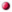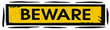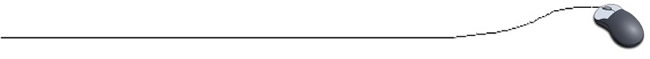Converting Strings to Numbers
and Numbers to Strings

 Strings to Numbers

Remember: numbers stored in strings must be converted to a numeric type
(such as an int) before they can be used in calculations.

Converting Strings to Numbers:
To convert a string value to a number, the following methods may be used:Convert using Integer.parseInt()
The parameter will be converted to a primitive int value. This is the most used method.
The chart below shows notations also for floats and doubles.
 Type Example int i = Integer.parseInt(String a); float f = Float.parseFloat(String a); double d = Double.parseDouble(String a);Example:
String numberString = "1701";
int number = Integer.parseInt(numberString);
System.out.println("The number is " + number + ".");

Output: The number is 1701.Convert using Integer.valueOf()

This method will return an Integer object, not a primitive int value.

Example:
String number = "20";
Integer convertedNumber = Integer.valueOf(number);
System.out.println("The number is " + number + ".");

Output: The number is 20.

Trying to convert String number = "twenty"; will cause a runtime error.
The computer has no idea that "twenty" is supposed to be 20.

Trying to convert String number = "10.5"; will also cause an error since it is not an integer.The conversions shown here are used to convert Strings (not characters) to numeric values. If you try to use these conversions on a character situation such as numberString.charAt(0) you will not get the desired result. When converting characters to number values, you need to use Character.getNumericValue().Numbers to Strings

Converting Numbers to Strings:
To convert a number value to a String, the following methods may be used:Convert an integer using Integer.toString()

This method is from the Integer class. This method will return a String object representing the int parameter. A popular method.

Example:
int number = 75;
String numberString = Integer.toString(number);

This result is equivalent to String numberString = "75";

The toString method can also be used with the conversion of a double.

String numberString = Double.toString(number);
The converted variable (numberString) will contain the String "75.0".Convert using String.valueOf(int)

This method is from the String class. This method will return an Integer object, not a primitive int value.

Example:
int number = 75;
String numberString = String.valueOf(number);

This result is equivalent to String numberString = "75";

This method may be applied to most primitive types.